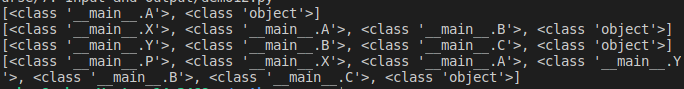# Method Resolution Order (MRO) in Python

## Method Resolution Order (MRO) in Python

In this article, I am going to discuss Method Resolution Order (MRO) in Python with examples. Please read our previous article where we discussed Inheritance in Python. As part of this article, we are going to discuss the following pointers which are related to Method Resolution Order in Python.

1. What is Method Resolution Order (MRO)
2. Three principles of MRO
##### Method Resolution Order (MRO)

In multiple inheritance scenarios, any specific attribute or method will initially be searched in the current class. If not found in the current class, then next search continues into parent classes in depth-first left to right fashion. Searching in this order is called Method Resolution Order (MRO).

##### Three principles of MRO:
1. The first principle is to search for the subclass before going for its base classes. If class B is inherited from A, it will search B first and then goes to A.
2. The second principle is, if any class is inherited from several classes, it searches in the order from left to right in the base classes. For example, if class C is inherited from A and B, syntactically class C(A, B), then first it will search in A and then in B.
3. The third principle is that it will not visit any class more than once. That means a class in the inheritance hierarchy is traversed only once exactly.

Understanding MRO gives you clear idea regarding which classes are being executed and in which sequence. We have a predefined method to see the sequence of execution of classes. It is: classname.mro()

##### Demo 1 for MRO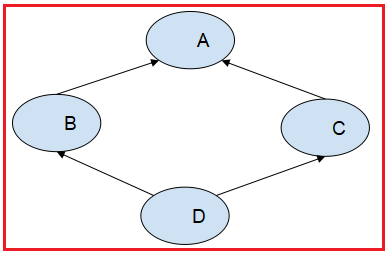Here,

1. mro(A)=A, object
2. mro(B)=B, A, object
3. mro(C)=C, A, object
4. mro(D)=D, B, C, A, object

Note: Object is a default super class in python.

###### Program: MRO (demo9.py)
```class A:
def m1(self):
print("m1 from A")
class B(A):
def m1(self):
print("m1 from B")
class C(A):
def m1(self):
print("m1 from C")
class D(B, C):
def m1(self):
print("m1 from D")

print(A.mro())
print(B.mro())
print(C.mro())
print(D.mro())```

Output: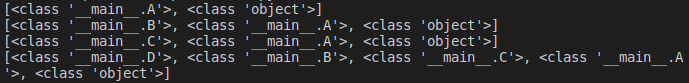###### Program: MRO contd (demo10.py)
```class A:
def m1(self):
print("m1 from A")
class B(A):
def m1(self):
print("m1 from B")
class C(A):
def m1(self):
print("m1 from C")
class D(B, C):
def m1(self):
print("m1 from D")

c=C()
c.m1()
print(C.mro())```

Output: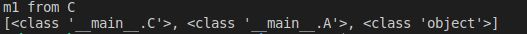In above program, with c object we are calling m1 method. So, m1() is already available in C class, so output is m1 from C.

###### Program: demo11.py
```class A:
def m1(self):
print("m1 from A")
class B(A):
def m1(self):
print("m1 from B")
class C(A):
def m2(self):
print("m2 from C")
class D(B, C):
def m1(self):
print("m1 from D")

c=C()
c.m1()
print(C.mro())```

Output: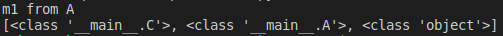In the above program, with the object ‘c’ we are calling the m1 method. But the m1() method is not available in class C. First the search will be in class C, then it will be in class A because A is super class to C. In class A, m1() method is available, so output is ‘m1 from A’.

###### Program: MRO contd. (demo12,py)
```class A:
def m1(self):
print("m1 from A")
class B(A):
def m1(self):
print("m1 from B")
class C(A):
def m1(self):
print("m1 from C")
class D(B, C):
def m1(self):
print("m1 from D")

d=D()
d.m1()
print(D.mro())```

Output: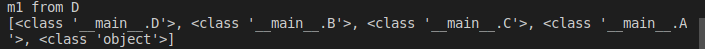In the above program, with object ‘d’ we are calling the m1 method. Since, method m1() is already available in class D, the output is ‘m1 from D’.

###### Program: MRO contd (demo13.py)
```class A:
def m1(self):
print("m1 from A")
class B(A):
def m1(self):
print("m1 from B")
class C(A):
def m1(self):
print("m1 from C")
class D(B, C):
def m3(self):
print("m3 from D")

d=D()
d.m1()
print(D.mro())```

Output: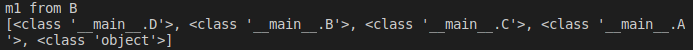In the above program, with object ‘d’ we are calling the method m1(). But, method m1() is not available in class D. So, next it will search in class B because it is super class to class D and also the first one to be searched according to MRO. In class B, m1() method is available, so output is ‘m1 from B’.

###### Program: MRO contd (demo14.py)
```class A:
def m1(self):
print("m1 from A")
class B(A):
def m2(self):
print("m1 from B")
class C(A):
def m1(self):
print("m1 from C")
class D(B, C):
def m3(self):
print("m3 from D")

d=D()
d.m1()
print(D.mro())```

Output: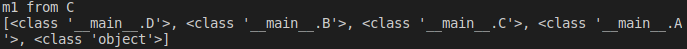In the above program, with object ‘d’ we are calling the method m1(). Since, method m1() is not available in class D, it will search in class B based on MRO (class B is superclass to class D). m1() method is also not available in class B. So, next it will search in class C because C is also super class to class D. In class C m1() method is available, so output is ‘m1 from C’.

###### Demo2 for MRO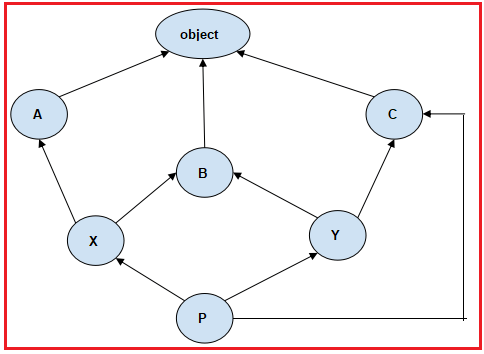Here,

1. mro(A)=A, object
2. mro(B)=B, object
3. mro(C)=C, object
4. mro(X)=X, A, B, object
5. mro(Y)=Y, B, C, object
6. mro(P)=P, X, A, Y, B, C, object
###### Program: MRO (demo15.py)
```class A:
def m1(self):
print("m1 from A")
class B:
def m1(self):
print("m1 from B")
class C:
def m1(self):
print("m1 from C")
class X(A, B):
def m1(self):
print("m1 from C")
class Y(B, C):
def m1(self):
print("m1 from A")
class P(X, Y, C):
def m1(self):
print("m1 from P")
print(A.mro())#AO
print(X.mro())#XABO
print(Y.mro())#YBCO
print(P.mro())#PXAYBCO```

Output: